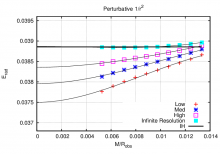# Rochester Institute of TechnologyPerturbative extraction of gravitational waveforms generated with Numerical Relativity
by H. Nakano, J. Healy, C.O. Lousto, Y. Zlochower
Published in Physical Review D 91, Issue 10, 104022 (Sunday, March 1, 2015)

## Abstract

We derive an analytical expression for extracting the gravitational waveforms at null infinity using the Weyl scalar ψ4 measured at a finite radius. Our expression is based on a series solution in orders of 1/r to the equations for gravitational perturbations about a spinning black hole. We compute this expression to order 1/r2 and include the spin parameter a of the Kerr background. We test the accuracy of this extraction procedure by measuring the waveform for a merging black-hole binary at ten different extraction radii (in the range r/M=75-190) and for three different resolutions in the convergence regime. We find that the extraction formula provides a set of values for the radiated energy and momenta that at finite extraction radii converges towards the expected values with increasing resolution, which is not the case for the `raw' waveform at finite radius. We also examine the phase and amplitude errors in the waveform as a function of observer location and again observe the benefits of using our extraction formula. The leading corrections to the phase are (1/r) and to the amplitude are (1/r2). This method provides a simple and practical way of estimating the waveform at infinity, and may be especially useful for scenarios such as well separated binaries, where the radiation zone is far from the sources, that would otherwise require extended simulation grids in order to extrapolate the `raw' waveform to infinity. Thus this method saves important computational resources and provides an estimate of errors.

## CCRG Authors

Healy, JamesLousto, Carlos O.Zlochower, Yosef

## Research Areas

Analytic Relativity & ModelingBinary Black Holes